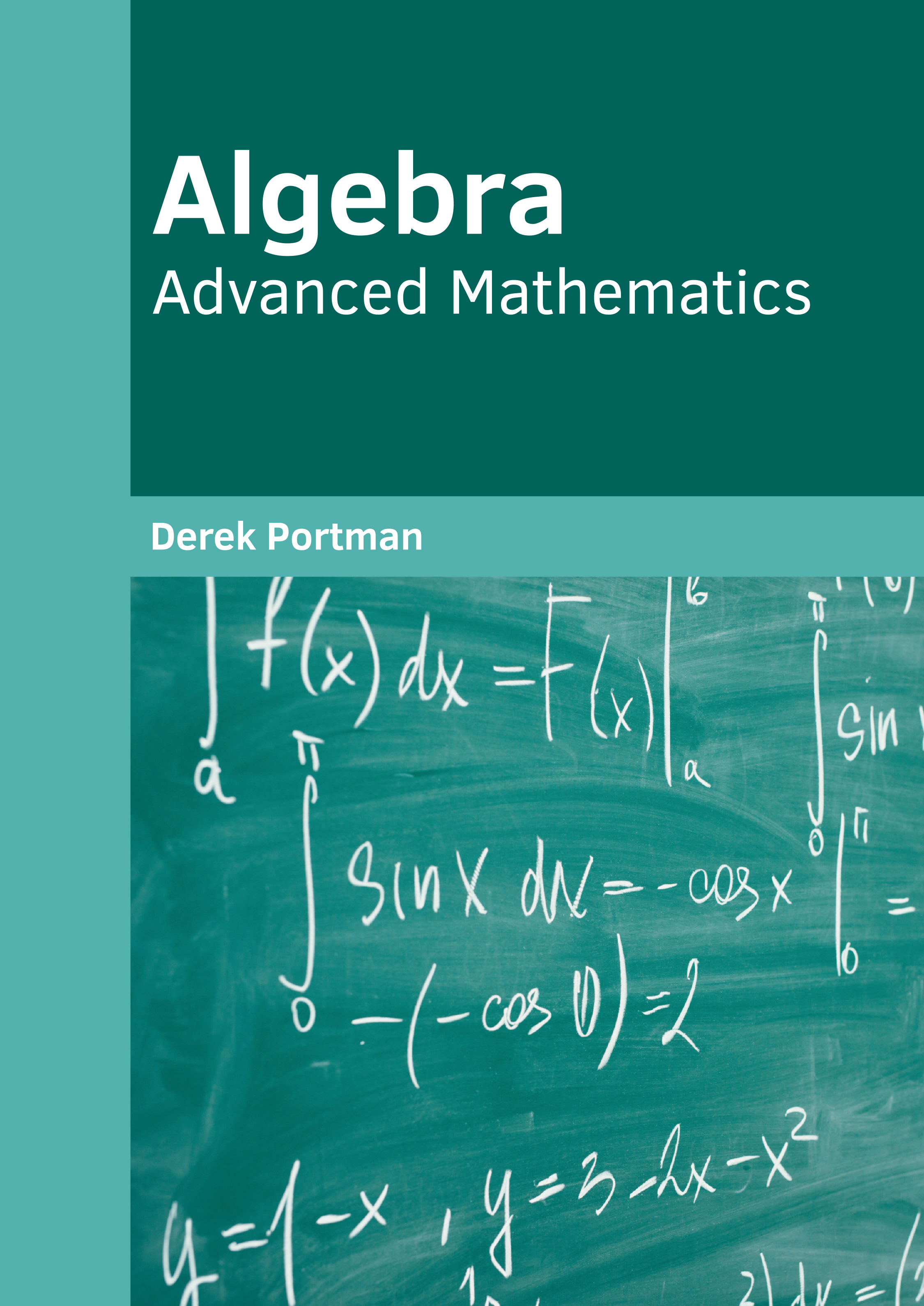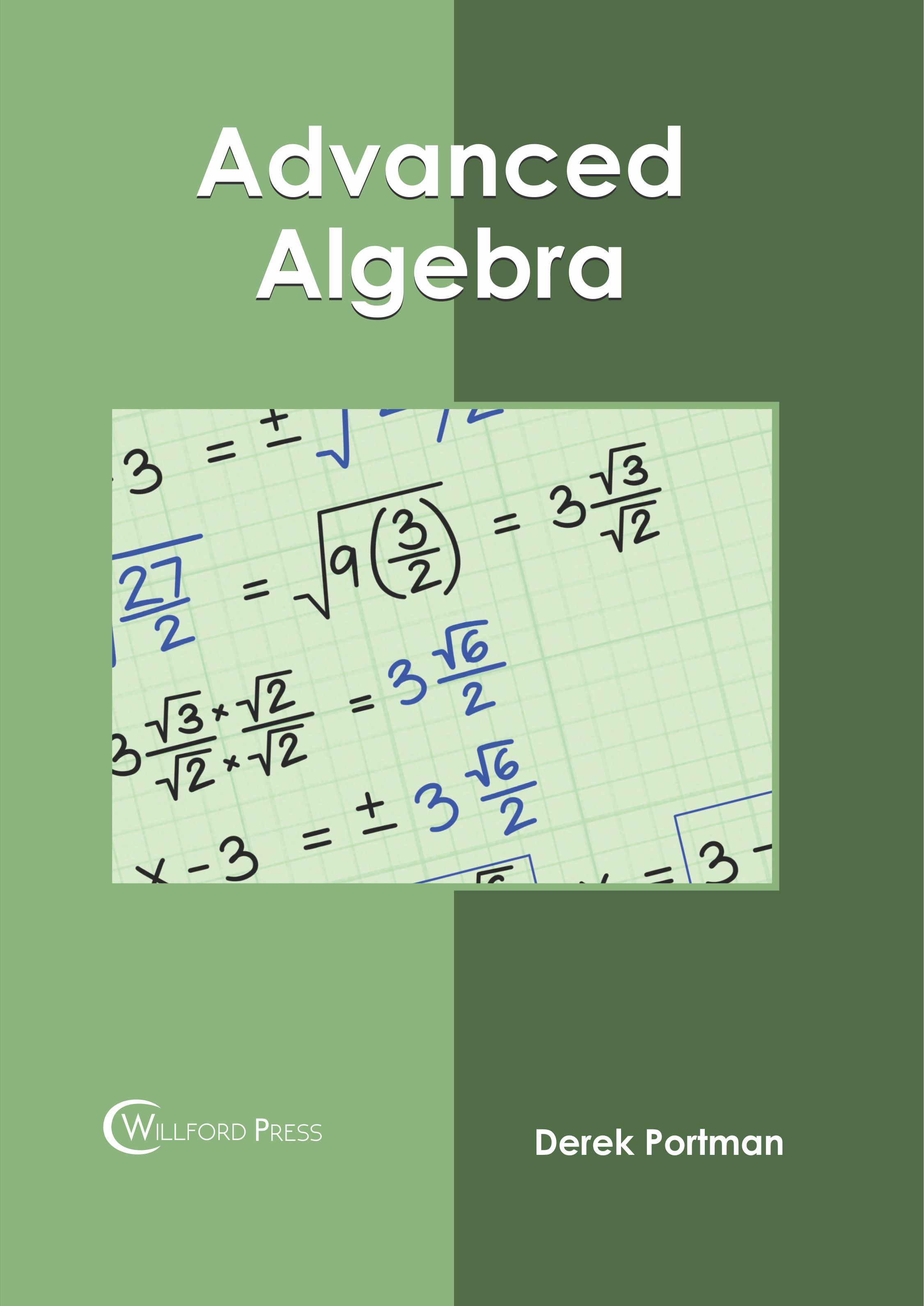BROWSE BY

#### Author

Results 1 - 3 of 3Algebra: Advanced Mathematics Author : Derek Portman Subject : Mathematics ISBN :9781682858257 Algebra is one of the major branches of mathematics which studies mathematical symbols and the rules used for handling these symbols. It deals with numerous concepts such as elementary equations and abstractions Read MoreAdvanced Algebra Author : Derek Portman Subject : Mathematics ISBN :9781682856345 Algebra is a sub-division of mathematics that involves elementary equation solving and study of abstractions. Advanced algebra or abstract algebra is the study of algebraic structures such as groups, rings, Read MoreAlgebra: Advanced Mathematics Author : Derek Portman Subject : Mathematics ISBN :9781647283469 Algebra is one of the major branches of mathematics which studies mathematical symbols and the rules used for handling these symbols. It deals with numerous concepts such as elementary equations and abstractions Read More
Results 1 - 3 of 3